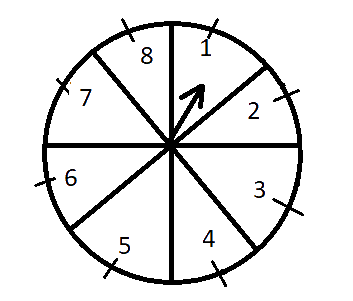# Ex.15.1 Q12 Probability Solution - NCERT Maths Class 10

Go back to  'Ex.15.1'

## Question

A game of chance consists of spinning an arrow which comes to rest pointing at one of the numbers $$1, 2, 3, 4, 5, 6, 7, 8$$ see Figure. and these are equally likely outcomes. What is the probability that it will point(i) at $$8$$?

(ii) an odd number?

(iii) a number greater than $$2$$?

(iv) a number less than $$9$$?

Video Solution
Probability
Ex 15.1 | Question 12

## Text Solution

What is known?

The arrow will come to rest pointing at one of the numbers $$1, 2, 3, 4, 5, 6, 7, 8$$ and these are equally likely outcomes. Totally there are $$8$$ outcomes.

What is unknown?

The probability that it will point:

(i) at $$8$$?

(ii) an odd number?

(iii) a number greater than $$2$$?

(iv) a number less than $$9$$?

Reasoning:

This question can be solved easily by using the formula

Probability of an event

$=\frac{\begin{bmatrix} \text { Number of}\\ \text{ possible outcomes }\end{bmatrix} }{ \begin{bmatrix}\text { Total no of} \\ \text{favorable outcomes} \end{bmatrix} }$

Steps:

(i) Total possible outcomes $$= 8$$

Probability of getting $$8$$ =

\begin{align}\frac{\text{Probability of getting }8}{\text{Total no of outcomes}}\end{align}

Probability of getting $$8$$ \begin{align}= \frac{1}{8}\end{align}

(ii)Total no of odd numbers$$=1,3,5,7 = 4$$

Probability of getting odd number \begin{align}&=\frac{\text{Total no of odd number}}{\text{Total no of outcomes}} \\ &=\frac{4}{8} \\ &=\frac{1}{2} \\\end{align}

(iii) Numbers greater than $$2$$ are $$3,4,5,6,7,8= 6$$

Probability of getting numbers greater than $$2$$

\begin{align}&=\frac{\text{Numbers greater than 2}}{\text{Total no of outcomes}} \\ &=\frac{6}{8} \\ &=\frac{3}{4} \\\end{align}

(iv) Numbers less than $$9$$ are $$1,2, 3,4,5,6,7,8= 8$$

Probability of getting numbers less than $$9$$

\begin{align}&=\frac{\text{ Numbers greater than 9}}{\text{Total no of outcomes}} \\& =\frac{8}{8}=1\end{align}

Learn from the best math teachers and top your exams

• Live one on one classroom and doubt clearing
• Practice worksheets in and after class for conceptual clarity
• Personalized curriculum to keep up with school